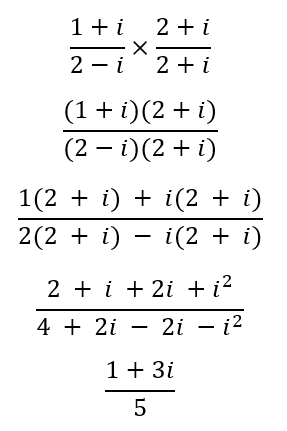# Multiplying and Dividing Complex Numbers Worksheets

How to Multiply and Divide Complex Numbers? When dealing with complex numbers, you have to be extra careful in resolving them. Especially if you have to multiply and divide them. Let us discuss both of these scenarios individually. Multiplication - Multiplying two or more complex numbers is similar to multiplying two or more binomials. Let us consider an example.
(3 + 2i)(2 - i)
Here, you will have to use the distributive property to write the above equation as:
3(2 - i) + 2i(2 - i)
6 - 3i + 4i - 2i2
Now, you must remember that i2=-1, thus, the equation becomes:
6 - 3i + 4i + 2
8+i
Division - Dividing complex numbers is just as simpler as writing complex numbers in fraction form and then resolving them. Let us consider an example:In this situation, the question is not in a simplified form; thus, you must take the conjugate value of the denominator.• ### Basic Lesson

Guides students solving equations that involve an Multiplying and Dividing Complex Numbers. Demonstrates answer checking. Example: Multiply: (5 - 4i)(7 - 3i)

• ### Intermediate Lesson

Demonstrates how to solve more difficult problems. Simplify: (6 - 8i)2

• ### Independent Practice 1

A really great activity for allowing students to understand the concept of Multiplying and Dividing Complex Numbers.

• ### Independent Practice 2

Students find the Multiplying and Dividing Complex Numbers in assorted problems. The answers can be found below.

• ### Homework Worksheet

Students are provided with problems to achieve the concepts of Multiplying and Dividing Complex Numbers.

• ### Skill Quiz

This tests the students ability to evaluate Multiplying and Dividing Complex Numbers.

Answers for math worksheets, quiz, homework, and lessons.

• ### Another Lesson

Guides students solving equations that involve an Multiplying and Dividing Complex Numbers. Demonstrates answer checking.

• ### Simplifying Lesson

Demonstrates how to solve more difficult problems.

• ### Independent Practice 3

A really great activity for allowing students to understand the concept of Multiplying and Dividing Complex Numbers.

• ### Independent Practice 4

Students find the Multiplying and Dividing Complex Numbers in assorted problems. The answers can be found below.

• ### Homework Worksheet 2

Students are provided with problems to achieve the concepts of Multiplying and Dividing Complex Numbers.

• ### Quiz 2

This tests the students ability to evaluate Multiplying and Dividing Complex Numbers.

• ### Lesson and Practice Answer Key

Answers for math lessons and practice sheets above.